Published: 01 December 2017

# Rheological and damping characteristics of adaptive materials for layered structures

Mikalai Zhurauski1
Algimantas Bubulis2
Evguenia Korobko3
Zoya Novikova4
1, 3, 4, 5Heat and Mass Transfer Institute of NASB, 220072, P. Brovka str. 15, Minsk, Belarus
2Kaunas University of Technology, LT-51424, Studentų str. 56, Kaunas, Lithuania
Corresponding Author:
Mikalai Zhurauski
Views 28

#### Abstract

Compositions of high-filled magnetically controlled materials with different dispersed phase particle sizes are developed. Rheological and damping characteristics of materials in the system of oscillations of three-layer plates are determined and their dependence on the size of magnetically sensitive particles is established.

## 1. Introduction

The need to use beams, plates and shells of a layered structure as constituent elements of thin-walled engineering constructions is due to the desire to obtain the lowest material capacity of products while maintaining the required strength and rigidity, and also to realize the possibility of changing their properties due to adaptive changes in their structure [1, 2].

To solve the problems of semi-active and active damping of vibrations, it is possible to use in thin-walled structures layers of materials that controllably change their viscoelastic properties under the influence of a magnetic field adaptively to the change in the dynamic state of a construction under external mechanical or thermal influences. Typically, such materials are based on the use of a high-viscosity or rubber-like matrix into which particles, for example, iron, orienting along the magnetic field vector are introduced [3, 4]. Performed experimental studies [5-8] show that almost all magnetically controlled materials exhibit a strong nonlinearity of their rheological properties as functions of magnetic field induction. The problem of creating composite plates based on magnetically controlled materials for use as controlled vibration protection elements reduces to the problem of optimal design in many parameters (geometric, physical), and also to the problem of optimal vibration suppression by means of magnetic field signals [9, 10].

We have developed compositions of magnetically sensitive disperse materials, which are highly filled pastes containing a complex dispersed phase consisting of two types of particles. Particles of the first type are sensitive to the influence of the magnetic field, of the second one form a thixotropic structural grid. The damping parameters of a three-layer “sandwich” plate with the use of dispersed materials as a viscoelastic layer, as well as rheological indexes of materials were experimentally investigated.

## 2. Materials and equipment

Particles of carbonyl iron were used as a component of a dispersed phase with high magnetorheological sensitivity, nanosized gamma-iron oxide particles $\gamma$-Fe2O3 were used as structure-forming agents. The composition of 4 samples of composite magnetically controlled materials of CMC (CMC-1, CMC-2, CMC-3, CMC-4), differing in the size of carbonyl iron particles is optimized. The concentration of carbonyl iron is 35 vol. %, of $\gamma$-Fe2O3 is 5.2 vol. %. The diameter of carbonyl iron particles was 3.5; 23; 13 and 1.17 µm respectively. The dispersion medium was a synthetic oil.

For rheological experiments, the rheometer Physica MCR 301 of Anton Paar (measuring cell is plate-plate type, plate diameter is 20 mm, gap is 0.7 mm) was used with a change in magnetic field induction $B$ up to 1 T. The measurements were carried out in 3 modes: 1) the mode of tangential shear of the upper plate with a constant shear rate in the range 0.01-100 s-1, which allowed obtaining flow curves; 2) the mode of linear growth of the shearing loading: strain curves are obtained and the values of the static yield stress ${\tau }_{0}$ are determined; 3) the mode of sinusoidal tangential shear oscillations with a constant frequency $f=$10 Hz and a deformation amplitude ${\gamma }_{a}$ in the range 0.0001-0.1 corresponding to the range of shear rate amplitude 0.0063-6.3 s-1. The components of the complex shear modulus ${G}^{*}=G\text{'}+iG\text{'}\text{'}$ (the storage modulus ${G}^{\text{'}}$ and the loss modulus $G\text{'}\text{'}$) were measured.

The damping characteristics of composite magnetically controlled materials when using in a “sandwich” plate as a viscoelastic layer under the action of a magnetic field of different intensity are determined in a laboratory experimental set-up for studying forced oscillations of plates. The set-up block diagram is shown in Fig. 1. The photo of the set-up is shown in Fig. 2.

Fig. 1Block diagram of the laboratory experimental set-up for studying forced oscillations of plates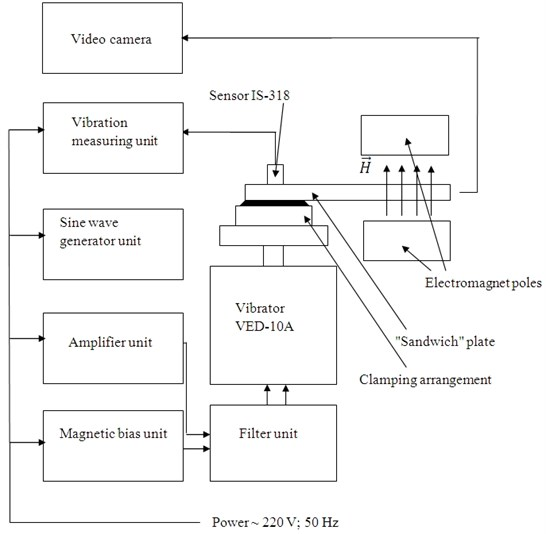Fig. 2Photo of the laboratory experimental set-up for studying forced oscillations of plates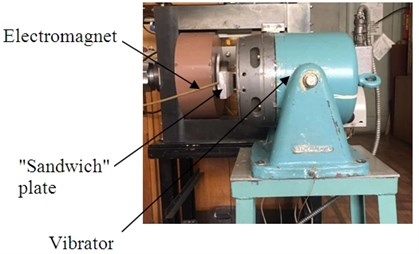The investigated “sandwich” plate with a length of 250 mm and a width of 20 mm, consisting of two aluminum plates and a magnetically controlled layer of 0.3 mm thick between them, is fixed by one end with a clamping arrangement on the vibrator table. The free end of the plate is placed between the poles of the electromagnet. The sine wave generator unit determines the vibrations of the vibrator's moving system and, accordingly, the clamped end of the plate. Acceleration of the clamped end of the sandwich is determined using a piezoelectric sensor and a vibration measuring unit. The amplitude of the oscillations of the free end of the plate is determined by means of a video camera connected to the computer. By varying the frequency of the forced oscillations and determining the corresponding amplitude of the oscillations, we obtain the amplitude-frequency characteristics of the “sandwich” plate. In the experiments, the frequency varied in the range 4-27 Hz. The intensity of the magnetic field between the poles of the electromagnet is set by the current in the windings of the pole pieces of the electromagnet, which is regulated by the power source of the electromagnet and is measured by an ammeter installed in the circuit.

## 3. Results and discussion

An example of a flow curve is shown in Fig. 3. It can be seen from the flow curves that the shear stress $\tau$ for a continuous flow increases by 2 orders of magnitude as the magnetic field induction increases. The flow curves of the materials studied are characteristic for nonlinear viscoplastic media. The greatest increase in $\tau$ occurs in the range of induction of a magnetic field of 500-1000 mT. A similar nature of the flow curves also exists for other samples. However, in this case, the absolute values of $\tau$ differ depending on the size of the carbonyl iron particles. Sample of CMC-4 containing smaller particles shows large values of shear stress at continuous flow and static yield stress in a magnetic field than CMC with coarse particles.

The dependence of the shear stress for continuous flow and the static yield stress on the induction of the magnetic field is showed in Fig. 4. The static yield stress is defined as the shear stress at which the structure of the material is destroyed. At a maximum field, the values of $\tau$ differ by more than 2 times. Probably, since at a diameter of carbonyl iron particles of 1.17 μm the size of the pores between the spherical iron particles is approximately the same as the size of $\gamma$-Fe2O3 (needle-shaped particles with lengths of 600 nm and 90 nm in thickness), this ensure a denser packing and, correspondingly, a stronger structure. A larger range of variation in rheological characteristics in a magnetic field suggests large damping indices.

Fig. 3Flow curves of composite magnetorheological material CMC-1 (diameter of carbonyl iron particles is 3.5 μm) in a magnetic field: 1 – magnetic field induction B= 0, 2 – B= 100 mT, 3-200, 4-300, 5-500, 6-1000.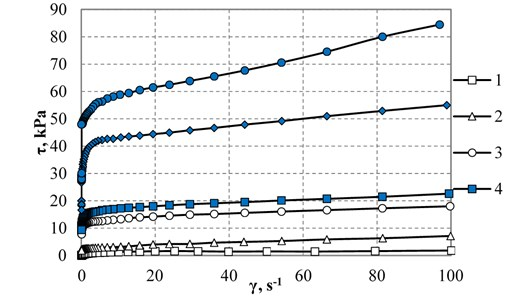The dependence of the components of the complex shear modulus of CMC on the strain amplitude in a magnetic field is shown in Fig. 5. The complex shear modulus characterizes the reaction of the material to non-stationary periodic influences. Its components ${G}^{\text{'}}$ and ${G}^{\text{'}\text{'}}$ grow quasi-linearly with magnetic field induction up to 0.2 mT, for large values of $B$${G}^{\text{'}}$ goes to saturation, and ${G}^{\text{'}\text{'}}$ decreases (in the linear region, at small deformations, where ${G}^{\text{'}}$ does not depend on $\gamma$). The components of the complex shear modulus practically do not depend on the particle size of the dispersed phase, in contrast to the shear stress at continuous flow and the static yield stress.

Fig. 4Dependences of the shear stress τ (γ= 15 s-1) a), the static yield stress τ0 b) of CMC samples containing carbonyl iron particles of different sizes on the magnetic field induction B: 1 – carbonyl iron particles size is 1.2 μm, 2 – 3.5 μm, 3-13, 4-23.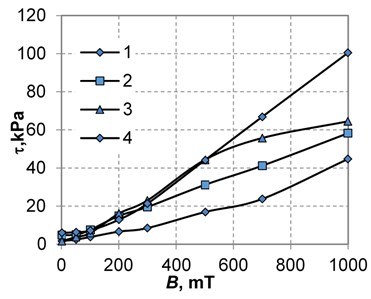a)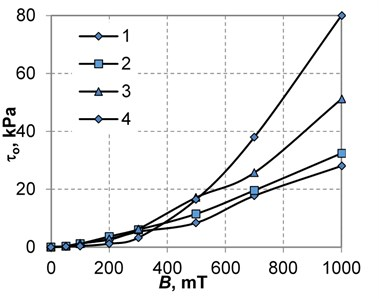b)

The amplitude-frequency characteristics of “sandwich” plates with a viscoelastic magnetically controlled layer based on CMC-1 (diameter of carbonyl iron particles is 3.5 μm) under forced oscillations are shown in Fig. 6. The resonance frequency of the plate is 14.5 Hz and is not significantly affected by the magnetic field strength and the size of the carbonyl iron particles. It can be seen that the amplitude of forced oscillations decreases in a magnetic field of intensity 300 kA/m by more than 2.5 times. Thus, plates with a magnetically controlled material show the ability to damping vibrations under magnetic field influence.

Fig. 5Dependence of the storage modulus a) and the loss modulus b) of CMC-1 with the carbonyl iron particle size of 3.5 μm on the shear strain amplitude: 1 – magnetic field induction B= 0, 2 – B= 100 mT, 3-200, 4-500, 5-1000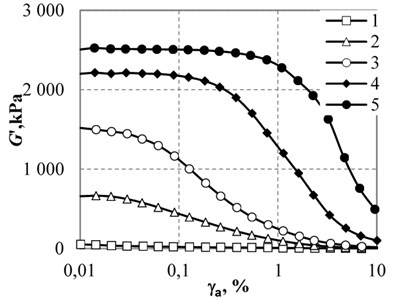a)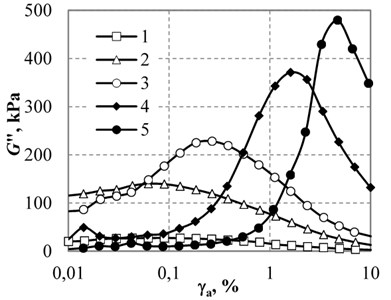b)

The dependence of the decrease in the amplitude of the oscillations at resonance (the ratio of the amplitude in the absence of a magnetic field to the amplitude of the oscillations in the magnetic field) on the size of the carbonyl iron particles is shown in Fig. 7.

Fig. 6The amplitude-frequency characteristics of “sandwich” plates with magnetically controlled material CMC-1: 1 – in the absence of a magnetic field, 2 – magnetic field strength is 100 kA/m, 3-200, 4-300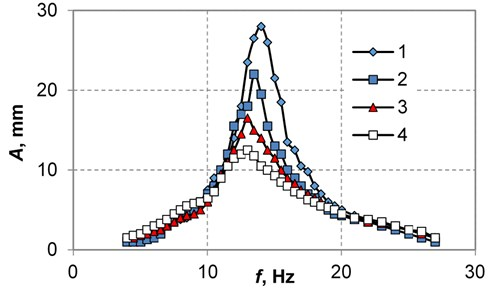Fig. 7The relative decrease in the resonance amplitude of forced oscillations of “sandwich” plates with a magnetically controlled material in a magnetic field as a function of the diameter of the carbonyl iron particles: 1 – magnetic field strength is 100 kA/m, 2-200, 3-300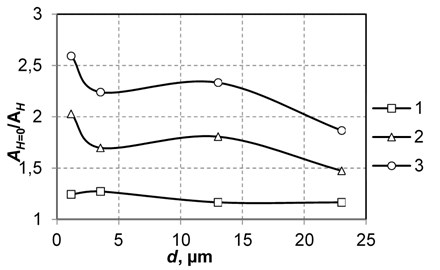Similar to the dependence of the shear stress on the particle size, where the greatest increase occurs when smaller particles are used, a greater damping is also achieved when using the CMC-4 material with the smallest particles.

## 4. Conclusions

Thus, when magnetorheological materials are used as a viscoelastic interlayer of layered plates, the amplitude of the forced oscillations of the plate is decreased at a resonance of more than 2.5 times. Dependence of damping characteristics of materials on the size of carbonyl iron particles is established: a more significant decrease in the amplitude with the use of smaller particles. The increase in rheological values (shear stress at continuous flow and static yield stress) of materials in a magnetic field also increases with decreasing particle size. The results obtained make it possible to consider promising the use of such controlled materials for the problem of damping of layered structures.

#### Cited by

Modeling of strain kinetics of damping viscoelastic magnetically controlled materials in creep mode